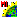# Query_NN.sml

###Download

#### Syntax Highlighing:

```comments, key words, predefined symbols, class members & methods, functions & classes
```
```#********************************************************************
#The following code prints out a list of the nearest
#neighbor polygons after querying the user for a vector object
#and a polygon number:
# QRY_NN.SML
# Given a single polygon of a vector object,
# print out the nearest neighbor polygons.
# AUTHOR: Paul Pope, Environmental Monitoring Program, UW-Madison
clear();
vector V;
# Prompt the user to enter the vector object
GetInputVector(V);
# Prompt the user to enter a polygon number of the vector object
numeric number_of_polygons = NumVectorPolys(V);
string prompt\$ = "Enter the polygon ID number";
numeric polygon_ID_number = PopupNum(prompt\$, 1, 1, number_of_polygons);
array numeric line_list;
array numeric nearest_neighbor_list;
numeric number_of_lines, number_of_neighbors;
number_of_lines = GetVectorPolyLineList(V,line_list, polygon_ID_number);
print("Number of Lines = ", number_of_lines);
number_of_neighbors = 0;
# Loop over the number of lines that make up the
# polygon and determine the nearest neighbor polygons
numeric j, k;
numeric leftpoly, rightpoly, outside_polygon, flag;
for j = 1 to number_of_lines step 1
{
print("Line Number = ", line_list[j]);
leftpoly = V.line[line_list[j]].Internal.LeftPoly;
rightpoly = V.line[line_list[j]].Internal.RightPoly;
print("Left Poly = ", leftpoly, "Right Poly = ", rightpoly);
# A nearest neighbor polygon will be either
# the leftpoly or the rightpoly to the line being evaluated
if (leftpoly != polygon_ID_number) then
{
outside_polygon = leftpoly;
}
if (rightpoly != polygon_ID_number) then
{
outside_polygon = rightpoly;
}
# Ensure that this nearest neighbor has not
# already been accounted for
flag = 0;
for k = 1 to number_of_neighbors step 1
{
if (nearest_neighbor_list[k] == outside_polygon) then
{
flag = 1;
}
}
# If this nearest neighbor has not been
# accounted for already, then add it to
# the list of nearest neighbors
if (flag != 1) then
{
# Make a copy of the nearest neighbor list
if (number_of_neighbors > 0) then
{
array numeric temp_list[number_of_neighbors];
for k = 1 to number_of_neighbors step 1
{
temp_list[k] = nearest_neighbor_list[k];
}
}
# Redefine the number of elements in the list
# of nearest neighbors and add the new nearest
# neighbor to the end of the list
number_of_neighbors = number_of_neighbors + 1;
array numeric nearest_neighbor_list[number_of_neighbors];
nearest_neighbor_list[number_of_neighbors] = outside_polygon;
# Copy all of the previously determined nearest
# neighbors into the newly expanded list
if (number_of_neighbors > 1) then
{
for k = 1 to (number_of_neighbors - 1) step 1
{
nearest_neighbor_list[k] = temp_list[k];
}
}
print("Nearest Neighbor = ", outside_polygon);
}  # End of if-then statement
}  # End of looping over j, the number of lines
# Clear the console and print out the list
# of nearest neighbors
clear();
print("For Polygon ID number = ", polygon_ID_number,", ");
print("the number of nearest neighbors = ", number_of_neighbors);
for k = 1 to number_of_neighbors step 1
{
print("Nearest Neighbor[", k, "] = ", nearest_neighbor_list[k]);
}
# End of SML script```